This example comes from a presentation by Qiqi Wang at Oxford in June 2013.

The logistic map is the iteration

$$x_{n+1} = r x_n (1-x_n),$$

where $r$ is a parameter in the interval $[0,4]$. The map behaves chaotically for certain larger values of $r$, and as $r$ increases, one has the classical example of period doubling as a route to chaos. A picture appears on the back cover of Strang's Introduction to Applied Mathematics .

Let's start our iteration with the constant value $x=0.5$, and see how it evolves for a range of values of $r$. Here are steps 0-3:

set(gcf, 'position', [0 0 600 420])
r = chebfun('r',[0 4]);
x = 0.5 + 0*r;
for n = 0:3
subplot(4,1,n+1)
plot(x), grid on, ylim([0 1])
ylabel(['x(' int2str(n) ')'])
text(.2,.7,['length(x) = ' int2str(length(x))])
x = r.*x.*(1-x);
end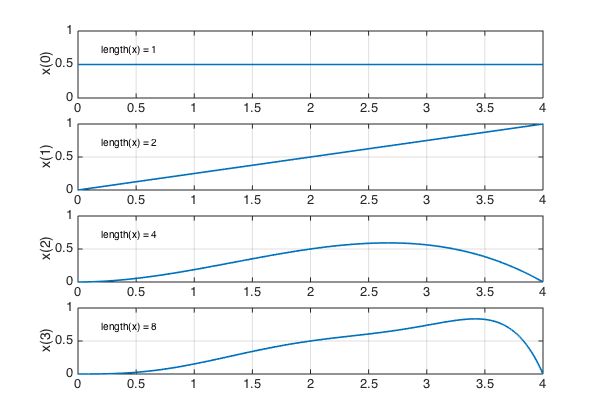Here are steps 4-7:

for n = 4:7
subplot(4,1,n-3)
plot(x), grid on, ylim([0 1])
ylabel(['x(' int2str(n) ')'])
text(.2,.7,['length(x) = ' int2str(length(x))])
x = r.*x.*(1-x);
end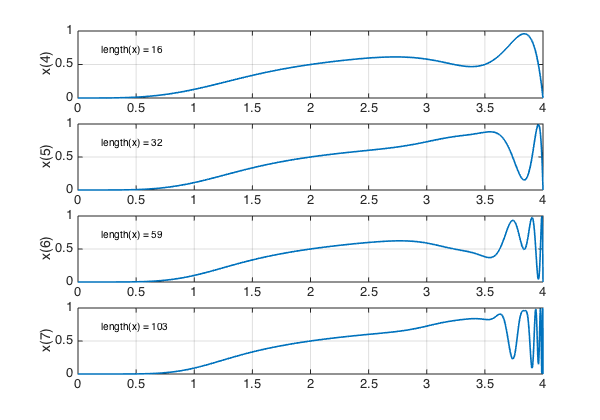Here are steps 8-11:

for n = 8:11
subplot(4,1,n-7)
plot(x), grid on, ylim([0 1])
ylabel(['x(' int2str(n) ')'])
text(.2,.7,['length(x) = ' int2str(length(x))])
x = r.*x.*(1-x);
end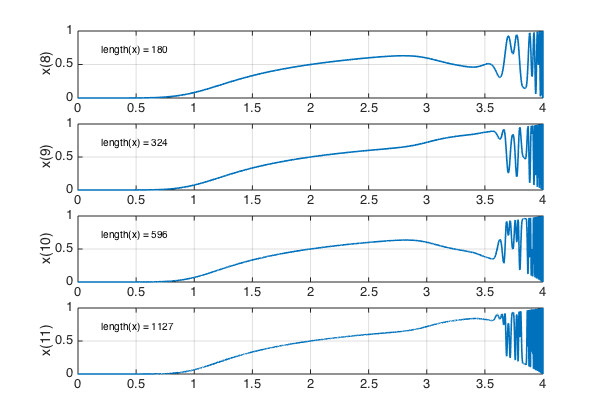Let's zoom in on the region $[3.5,4]$ and look at steps 12-15:

r = r{3.5,4}; x = x{3.5,4};
for n = 12:15
subplot(4,1,n-11)
plot(x), grid on, ylim([0 1])
ylabel(['x(' int2str(n) ')'])
text(3.52,.7,['length(x) = ' int2str(length(x))])
x = r.*x.*(1-x);
end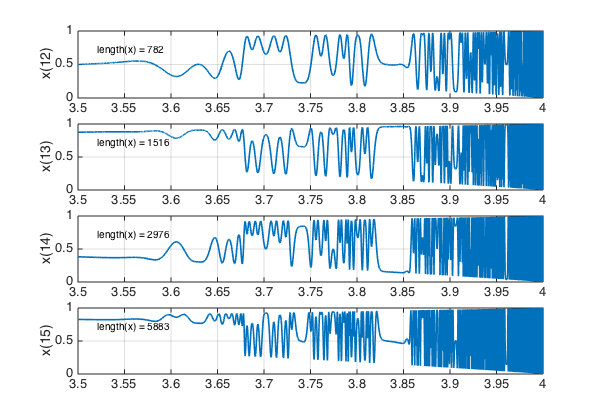And here are steps 16-18:

for n = 16:18
subplot(4,1,n-15)
plot(x), grid on, ylim([0 1])
ylabel(['x(' int2str(n) ')'])
text(3.52,.7,['length(x) = ' int2str(length(x))])
x = r.*x.*(1-x);
end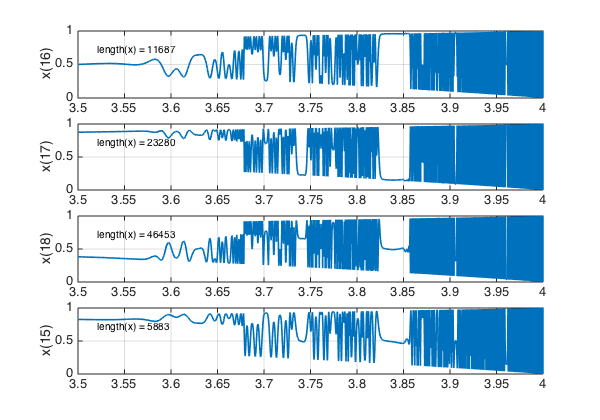The reader can have some fun examining these pictures. Where do we see period 1, period 2, period 4, chaos? How does this match what is known about dependence on $r$?

Let's see the final plot more fully:

figure
plot(x), ylim([0 1]), grid on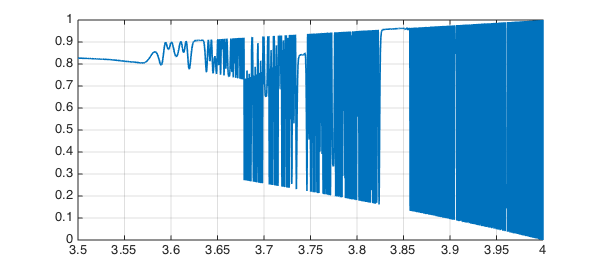And let's zoom in on a small interval:

plot(x,'interval',[3.902,3.908]), ylim([0 1]), grid on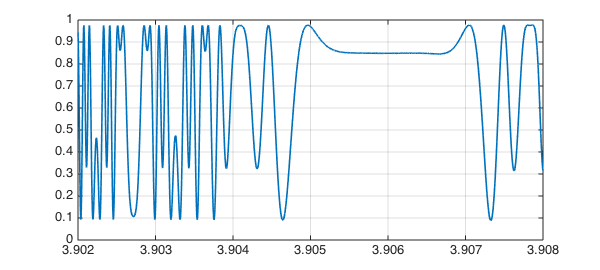1. G. Strang, Introduction to Applied Mathematics, Wellesley-Cambridge Press, 1986.